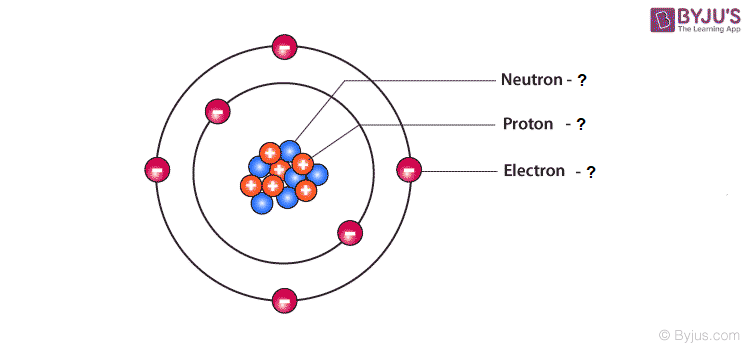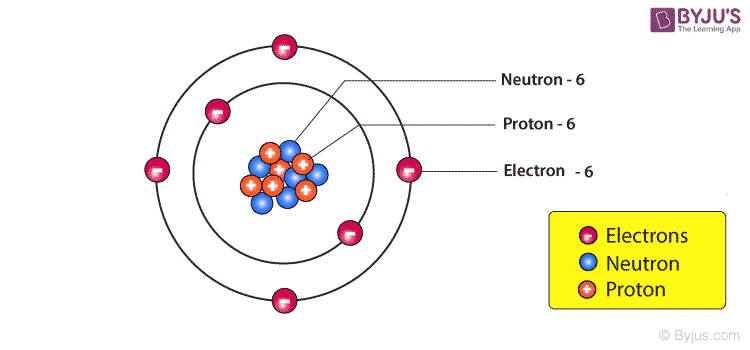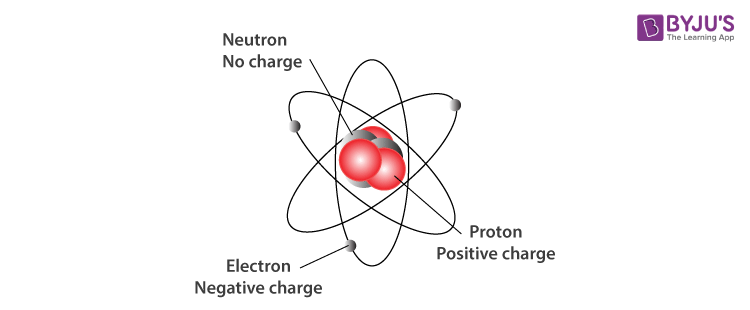Jet Set Go! All about Aeroplanes Jet Set Go! All about Aeroplanes

# Sub Atomic Particles Questions

In chemistry, a sub-atomic particle is a particle that comprises an atom. Electrons, protons and neutrons collectively are known as the sub-atomic particles that form an atom. An electron is a negatively charged sub-atomic particle that revolves around the nucleus, whereas Proton is a positively charged sub-atomic particle. On the other hand, a neutron is a neutral sub-atomic particle. James Chadwick was the first to discover the presence of neutrons inside an atom in 1932. Neutrons and protons are collectively known as nucleons.

 Definition: Subatomic particles are the particles that constitute an atom. Electrons, protons and neutrons collectively are known as the sub-atomic particles that constitute an atom.

## Sub Atomic Particles Chemistry Questions with Solutions

Q1. Which of the following has a positive charge?

(a) Electrons

(b) Protons

(c ) Neutrons

(d) None of the above

Answer: (b) Proton has a positive charge.

Q2. Neutrons and protons are collectively known as

(a) Nucleons

(b) Mass number

(c ) Atomic number

(d) None of the above

Answer: (a) Neutrons and protons are collectively known as nucleons.

Q3. The number of protons in an atom is equivalent to the number of

(a) Neutrons

(b) Protons

(c ) Electrons

(d) None of the above

Answer: (c ) The number of protons in an atom is equivalent to the number of electrons.

Q4. Name the scientist who was first to propose the atomic theory of an atom?

(a) J. J. Thomson

(b) John Dalton

(c ) Neils Bohr

(d) None of the above

Answer: (b) John Dalton was the first to propose the atomic theory of an atom.

Q5. What was the source of alpha particles in the Rutherford scattering experiment?

(a) Hydrogen nucleus

(b) Helium nucleus

(c ) Lithium nucleus

(d) None of the above

Answer: (b) Helium nucleus was the source of alpha particles in the Rutherford scattering experiment.

Q6. Is anything smaller than an atom?

Answer: The sub-atomic particles are smaller than an atom. Electrons, protons and neutrons collectively are known as the sub-atomic particles that constitute an atom.

Q7. What is an electron?

Answer: Electron is an atom’s negatively charged sub-atomic particle that revolves around the nucleus. J. Thompson was the first to discover the presence of electrons inside an atom.

Q8. What is a proton?

Answer: Proton is an atom’s positively charged sub-atomic particle. Ernest Rutherford was the first to discover the presence of electrons inside an atom.

Q9. What is a neutron?

Answer: A neutron is an atom’s neutral sub-atomic particle. James Chadwick was the first to discover the presence of neutrons inside an atom in 1932. Neutrons and protons are collectively known as nucleons.

Q10. Identify the number of electrons, protons and neutrons in the figure mentioned below.Answer: The figure mentioned above has six electrons, protons and neutrons.Q11. Identify the subatomic particles (electrons, protons and neutrons) in the following elements.

(a) α

(b) 2H1

Answer: (a) Alpha particle is a helium atom (4H2).

The total number of electrons = 2

The total number of protons = 2

The total number of neutrons = Mass number – Atomic number

The total number of neutrons = 4 – 2

The total number of neutrons = 2.

(b) 2H1 is an isotope of hydrogen known as deuterium.

The total number of electrons = 1,

The total number of protons = 1,

and The total number of neutrons = Mass number – Atomic number.

The total number of neutrons = 2 – 1.

The total number of neutrons = 1.

Q12. Fill in the table.

Atomic number

Mass number

Number of protons

Number of electrons

Number of neutrons

2

2

23

11

15

16

85

37

53

74

Atomic number

Mass number

Number of protons

Number of electrons

Number of neutrons

2

4

2

2

2

11

23

11

12

11

15

31

15

16

15

37

85

37

48

37

53

127

53

74

53

Q13. Name the three sub-atomic particles of an atom.

Answer: The three sub-atomic particles of an atom are mentioned below.

1. Electrons

2. Protons

3. Neutrons.Q14. What is the limitation of Dalton’s Atomic Theory?

Answer: John Dalton was the first to explain the structure of an atom. However, he was unable to explain the structure of an atom correctly. A few shortcomings of Dalton’s Atomic Theory are mentioned below.

1. John Dalton’s theory does not account for subatomic particles.

2. He failed to explain the existence of isotopes.

3. He failed to explain the existence of isobars.

4. He failed to explain the existence of allotropes.

5. He failed to explain the existence of fractional ratio compounds such as CaSO4. ½ H2O.

Q15. How do the atomic structures of an isotope range?

Answer: The atomic structures of an isotope range in terms of the number of nucleons.

## Practise Questions on Sub Atomic Particles

Q1. What are the postulates of Dalton’s Atomic Theory?

Q2. What are the limitations of Bohr’s atomic theory?

Q3. How did Sommerfeld modify Bohr’s theory?

Q4. What is the significance of Rutherford’s atomic model?

Q5. What are the postulates of the Rutherford Atomic Model?

Click the PDF to check the answers for Practice Questions.

## Structure of Atom Class 11 Chemistry## Rutherford’s Model, J.J Thomson Model – Structure of Atom## Atomic Structure Class 11 Chemistry## Structure of Atom Class 11 Chemistry## Structure of Atom Class 11 One Shot (Full Chapter Revision)## Top 12 Most Important Questions of Structure of Atom Class 11 Chemistry for JEE Mains 2022